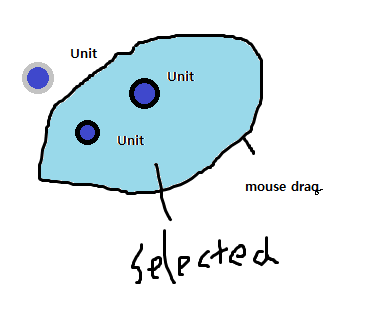# How to make free area selection(like lasso tool in photoshop) in rts?

How to make free area selection drawn by mouse(like lasso tool in photoshop) for objects in rts game?i can make selection box, but i dont know how to make this.

You can detect objects inside an arbitrary region in Unity with the following trick: create a mesh trigger with the desired shape, add a kinematic rigidbody and force collision calculation by “moving” the trigger to its own position: OnTriggerEnter events will be generated for each collider inside the trigger, and you can collect them in a list or set a flag in each object’s script.

The example below generates a squared horizontal mesh collider measuring 5x5 and prints the names of all objects detected. Attach this script to an empty object placed at the center of the area you want to check:

``````#pragma strict

private var vertices: Vector3[] = [
Vector3(-5,0,0),
Vector3(0,0,5),
Vector3(5,0,0),
Vector3(0,0,-5)
];

private var tris: int[] = [
0,1,2,
2,3,0
];

function DetectRegion(){
var mesh: Mesh = new Mesh(); // create a mesh...
mesh.vertices = vertices; // with the desired shape
mesh.triangles = tris;
col.isTrigger = true;
col.sharedMesh = mesh;
col.convex = true;
// and a kinematic rigidbody
rb.isKinematic = true;
// force collision calculation:
rigidbody.position = transform.position;
// wait next FixedUpdate...
yield WaitForFixedUpdate();
// and destroy the trigger
Destroy(rb);
Destroy(col);
Destroy(mesh);
}

function OnTriggerEnter(other: Collider){
// one OnTriggerEvent is generated for each object
print(other.name);
}

function Update(){
// detect objects inside region when D is pressed:
if (Input.GetKeyDown("d")) DetectRegion();
}
``````

Now comes the worst part: track the mouse movement and generate a mesh with these points. This is a very complicated task, and I suggest you to post another question about this particular subject - something like "How to create an arbitrary horizontal mesh with the mouse?
"

C# version (haven’t tested it)

``````    Vector3[] edgeVerts;
Vector3[] vertices;
int[] tris;
int pointSpacing = 5;
Vector3 mousePos;
List<Vector3> edges = new List<Vector3>();
int maxDist = 100;

void Update () {
if(Input.GetMouseButtonDown(0)){
//Array.Clear(edges, 0, edges.Length);
edges.Clear();
SaveEdge();
}
if(Input.GetMouseButton(0)){
if((mousePos-Input.mousePosition).sqrMagnitude >= pointSpacing*pointSpacing){
SaveEdge();
}
}
if(Input.GetMouseButtonUp(0)){
tris = CreateTris();
CreateMesh(edges.ToArray(), tris);
}
}

void SaveEdge () {
mousePos = Input.mousePosition;
}

int[] CreateTris() {
List<int> trisList = new List<int>();
for(var i = 0; i < edges.Count; i++){
}
return trisList.ToArray();
}

IEnumerator  CreateMesh (Vector3[] verts, int[] tris) {
Mesh mesh = new Mesh();
mesh.vertices = verts;
mesh.triangles = tris;

col.isTrigger = true;
col.sharedMesh = mesh;
col.convex = true;